# 1. Independent Component Analysis (ICA)

Department of Artificial Intelligence Medicine, Graduate School of Medicine, Chiba University

# Introduction

In this vignette, we consider approximating a data matrix as a product of multiple low-rank matrices (a.k.a., factor matrices).

Test data is available from toyModel.

library("iTensor")
data1 <- iTensor::toyModel("ICA_Type1")
data2 <- iTensor::toyModel("ICA_Type2")
data3 <- iTensor::toyModel("ICA_Type3")
data4 <- iTensor::toyModel("ICA_Type4")
data5 <- iTensor::toyModel("ICA_Type5")
dim(data1$X_observed) ##  500 3 dim(data2$X_observed)
##  500   3
dim(data3$X_observed) ##  500 3 dim(data4$X_observed)
##  480   3
dim(data5$gene) # N < P systems ##  64 3116 Summary of these data is as follows: 1. ICA with time-independent sub-Gaussian data 2. ICA with time-independent super-Gaussian data 3. ICA with data mixed with signals having no time dependence and different kurtosis 4. ICA with time-dependent data 5. IPCA in N < P systems You will see that the stuctures of these data as follows. pairs(data1$X_observed, main="data1")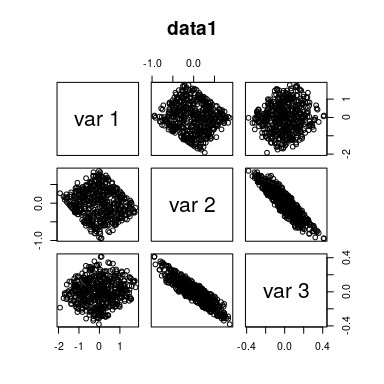pairs(data2$X_observed, main="data2")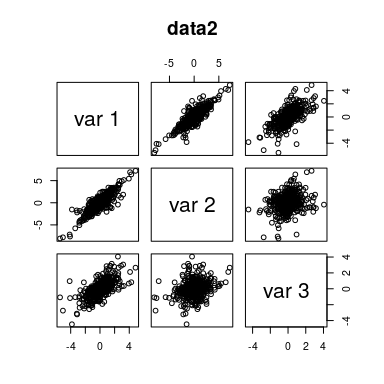pairs(data3$X_observed, main="data3")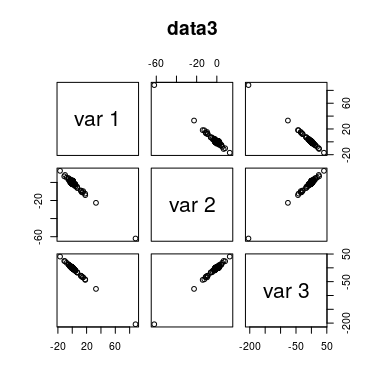pairs(data4$X_observed, main="data4")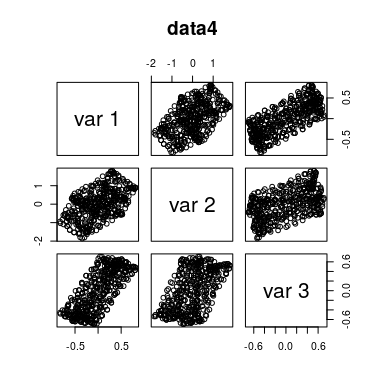dim(data5$gene)
##    64 3116

To perform and visualize all the ICA at once, here we write some functions as follows.

allICA <- function(X, J){
# Classic ICA Methods
out.FastICA <- ICA(X=X, J=J, algorithm="FastICA")
out.InfoMax <- ICA(X=X, J=J, algorithm="InfoMax")
out.ExtInfoMax <- ICA(X=X, J=J, algorithm="ExtInfoMax")
# Modern ICA Method
# Output
list(out.FastICA=out.FastICA, out.InfoMax=out.InfoMax,
}

CorrIndexAllICA <- function(S, out){
tmp <- lapply(out, function(x, S){CorrIndex(cor(S, Re(x$S)))}, S=S) Name <- gsub("out.", "", names(tmp)) Value <- round(unlist(tmp), 3) names(Value) <- NULL cbind(Name, Value) tmp <- as.data.frame(cbind(Name, Value)) colnames(tmp) <- c("Method", "CorrIndex") knitr::kable(tmp) } Note that we select only four ICA algorithms for the demonstration but in iTensor 12 ICA algorithms are available. For the details, check ?ICA and ?ICA2. # 1. ICA with time-independent sub-Gaussian data In this data, according to the independence between estimated source signal, an upright square means that the calculation is successful and other cases such as a rhombus rotated 45 degrees from a square means that the calculation is fail. set.seed(123456) out1 <- allICA(X=data1$X_observed, J=3)

Here we use CorrIndex, which is a performance index to evaluate ICA results (Sobhani 2022). CorrIndex imply that FastICA, ExtInfoMax, and JADE performed well (the closer to 0, the better the performance).

CorrIndexAllICA(data1$S_true, out1) Method CorrIndex FastICA 0.263 InfoMax 7.439 ExtInfoMax 0.263 JADE 0.221 We can see the details of source signals as follows. pairs(out1$out.FastICA$S, main="FastICA")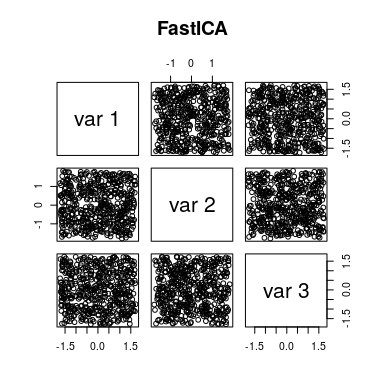pairs(out1$out.InfoMax$S, main="InfoMax")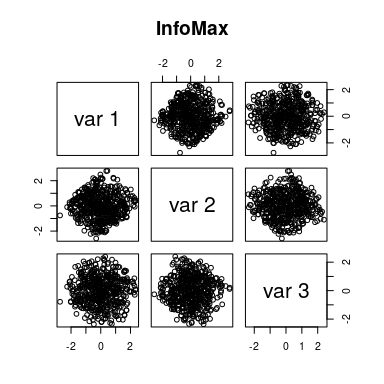pairs(out1$out.ExtInfoMax$S, main="ExtInfoMax")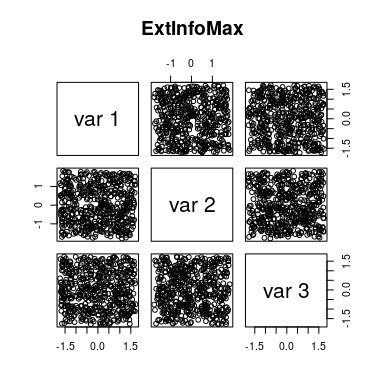pairs(Re(out1$out.JADE$S), main="JADE")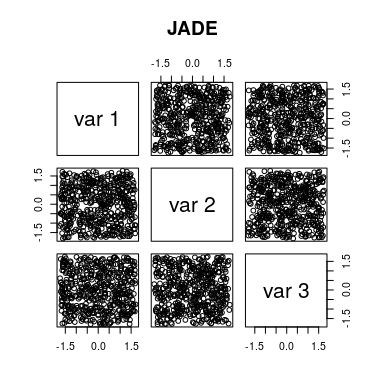# 2. ICA with time-independent super-Gaussian data In this data, if the outliers are distributed along the lines of x = 0 and y = 0, the calculation is successful and if they are of the cross-shape, it is a failure. set.seed(123456) out2 <- allICA(X=data2$X_observed, J=3)

CorrIndex imply that all the algorithms performed well.

CorrIndexAllICA(data2$S_true, out2) Method CorrIndex FastICA 0.268 InfoMax 0.322 ExtInfoMax 0.268 JADE 0.374 We can see the details of source signals as follows. pairs(out2$out.FastICA$S, main="FastICA")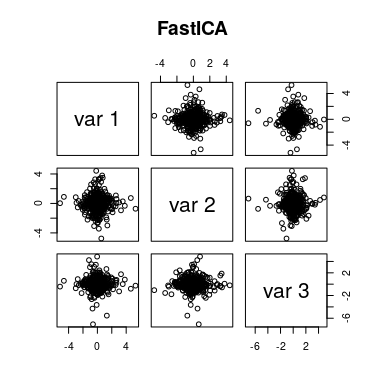pairs(out2$out.InfoMax$S, main="InfoMax")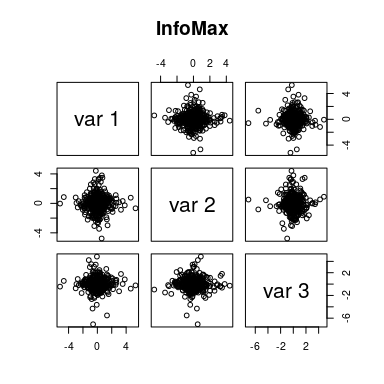pairs(out2$out.ExtInfoMax$S, main="ExtInfoMax")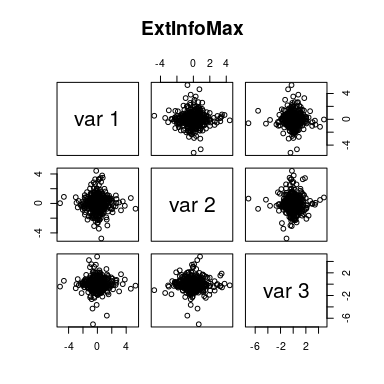pairs(Re(out2$out.JADE$S), main="JADE")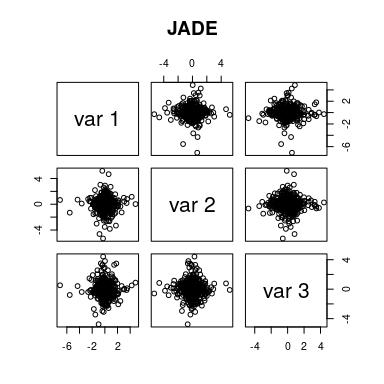# 3. ICA with data mixed with signals having no time dependence and different kurtosis In this data, the uniform distribution should be extracted in such a way that it is not rhombic, and furthermore the normal distribution and the t-distribution with 1 degree of freedom should be extracted independently. Sometimes, it is difficult ot determine visually though. set.seed(123456) out3 <- allICA(X=data3$X_observed, J=3)

CorrIndex imply that FastICA, ExtInfoMax, and JADE performed well.

CorrIndexAllICA(data3$S_true, out3) Method CorrIndex FastICA 0.23 InfoMax 3.799 ExtInfoMax 0.213 JADE 0.408 We can see the details of source signals as follows. pairs(out3$out.FastICA$S, main="FastICA")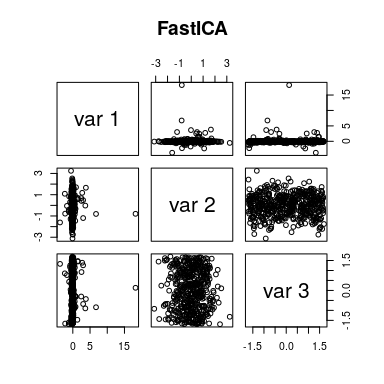pairs(out3$out.InfoMax$S, main="InfoMax")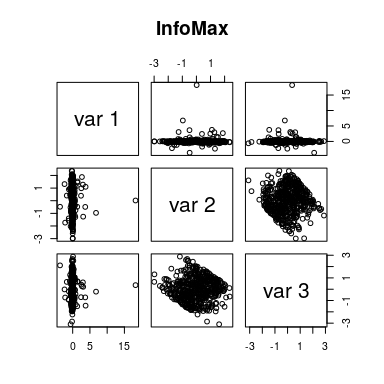pairs(out3$out.ExtInfoMax$S, main="ExtInfoMax")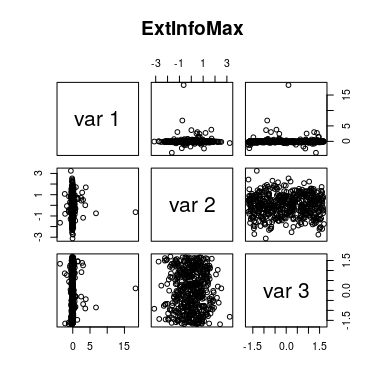pairs(Re(out3$out.JADE$S), main="JADE")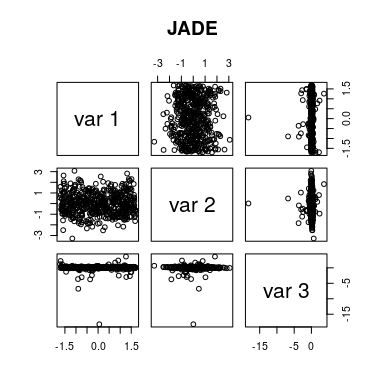# 4. ICA with time-dependent data In this data, if the mesh pattern is reproduced, the calculation is successful. set.seed(123456) out4 <- allICA(X=data4$X_observed, J=3)

CorrIndex imply that FastICA, ExtInfoMax, and JADE performed well.

CorrIndexAllICA(data4$S_true, out4) Method CorrIndex FastICA 0.081 InfoMax 8.026 ExtInfoMax 0.081 JADE 0.08 We can see the details of source signals as follows. pairs(out4$out.FastICA$S, main="FastICA")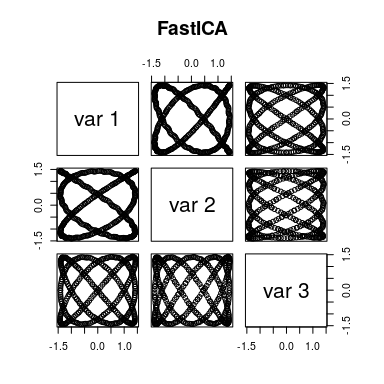pairs(out4$out.InfoMax$S, main="InfoMax")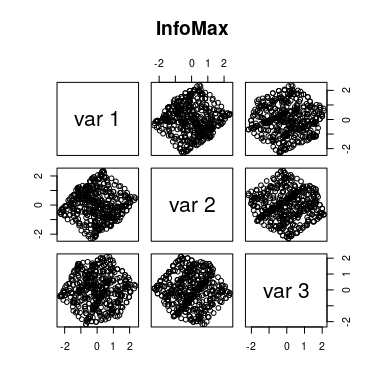pairs(out4$out.ExtInfoMax$S, main="ExtInfoMax")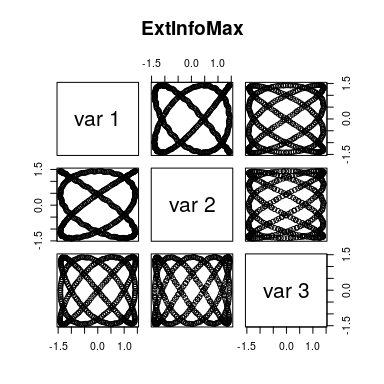pairs(Re(out4$out.JADE$S), main="JADE")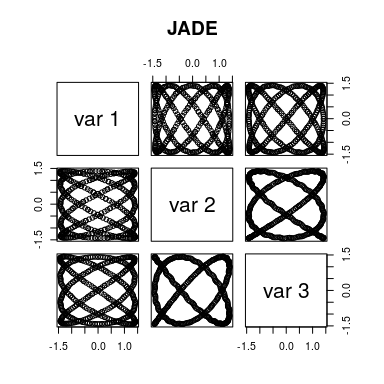# 5. IPCA in N < P systems This kind of data is an thin and long matrix, which is a very common data structure in omics data. In iTensor, we re-implemented the IPCA function of mixOmics package. library("mixOmics") ## Loading required package: MASS ## Loading required package: lattice ## Loading required package: ggplot2 ## ## Loaded mixOmics 6.24.0 ## Thank you for using mixOmics! ## Tutorials: http://mixomics.org ## Bookdown vignette: https://mixomicsteam.github.io/Bookdown ## Questions, issues: Follow the prompts at http://mixomics.org/contact-us ## Cite us: citation('mixOmics') set.seed(123456) # mixOmics's IPCA res.ipca <- ipca(data5$gene, ncomp=3, mode="deflation")

# iTensor's IPCA
out5 <- ICA2(X=as.matrix(data5$gene), J=3, algorithm="IPCA") We can see the details of source signals as follows. In this data, we can confirm that the IPCA of mixOmics and iTensor have the same results, except for the order and constant-fold relationships. pairs(res.ipca$x, main="IPCA (mixOmics)", col=data5$treatment[,"Treatment.Group"], pch=16)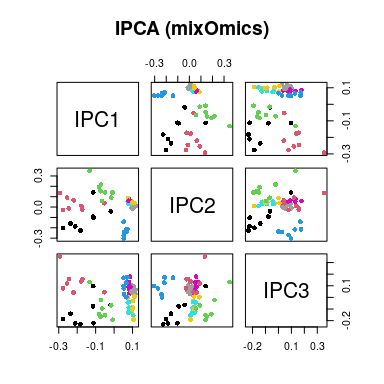pairs(out5$S, main="IPCA (iTensor)", col=data5\$treatment[,"Treatment.Group"], pch=16)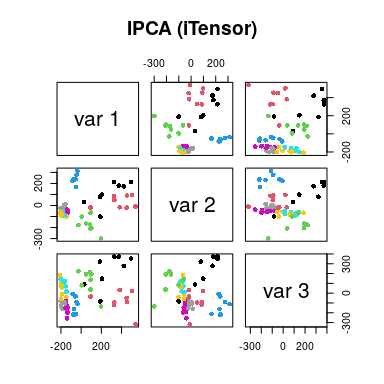# Session Information

## R version 4.3.0 (2023-04-21)
## Platform: x86_64-pc-linux-gnu (64-bit)
## Running under: Debian GNU/Linux bookworm/sid
##
## Matrix products: default
## LAPACK: /usr/lib/x86_64-linux-gnu/openblas-pthread/libopenblasp-r0.3.21.so;  LAPACK version 3.11.0
##
## locale:
##   LC_CTYPE=en_US.UTF-8       LC_NUMERIC=C
##   LC_TIME=en_US.UTF-8        LC_COLLATE=en_US.UTF-8
##   LC_MONETARY=en_US.UTF-8    LC_MESSAGES=en_US.UTF-8
##   LC_PAPER=en_US.UTF-8       LC_NAME=C
##  LC_MEASUREMENT=en_US.UTF-8 LC_IDENTIFICATION=C
##
## time zone: Etc/UTC
## tzcode source: system (glibc)
##
## attached base packages:
##  stats     graphics  grDevices utils     datasets  methods   base
##
## other attached packages:
##  mixOmics_6.24.0 ggplot2_3.4.2   lattice_0.21-8  MASS_7.3-59
##  iTensor_1.0.2
##
## loaded via a namespace (and not attached):
##   tidyr_1.3.0         sass_0.4.5          utf8_1.2.3
##   generics_0.1.3      stringi_1.7.12      digest_0.6.31
##   magrittr_2.0.3      evaluate_0.20       grid_4.3.0
##  RColorBrewer_1.1-3  fastmap_1.1.1       plyr_1.8.8
##  jsonlite_1.8.4      Matrix_1.5-4        ggrepel_0.9.3
##  RSpectra_0.16-1     gridExtra_2.3       mgcv_1.8-42
##  purrr_1.0.1         fansi_1.0.4         scales_1.2.1
##  codetools_0.2-19    jquerylib_0.1.4     cli_3.6.1
##  rlang_1.1.0         splines_4.3.0       munsell_0.5.0
##  withr_2.5.0         cachem_1.0.7        yaml_2.3.7
##  ellipse_0.4.5       tools_4.3.0         parallel_4.3.0
##  reshape2_1.4.4      BiocParallel_1.34.0 einsum_0.1.0
##  dplyr_1.1.2         colorspace_2.1-0    corpcor_1.6.10
##  groupICA_0.1.1      vctrs_0.6.2         R6_2.5.1
##  matrixStats_0.63.0  lifecycle_1.0.3     stringr_1.5.0
##  pkgconfig_2.0.3     rTensor_1.4.8       geigen_2.3
##  pillar_1.9.0        bslib_0.4.2         gtable_0.3.3
##  jointDiag_0.4       glue_1.6.2          rARPACK_0.11-0
##  Rcpp_1.0.10         highr_0.10          xfun_0.39
##  tibble_3.2.1        tidyselect_1.2.0    knitr_1.42
##  nlme_3.1-162        htmltools_0.5.5     igraph_1.4.2
##  rmarkdown_2.21      compiler_4.3.0